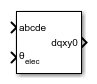# Coordinate Transform (Five-Phase)

Implement abcde to dqxy0 transform

• Library:
• Simscape / Electrical / Control / Mathematical Transforms

•## Description

The Coordinate Transform (Five-Phase) block converts the time-domain components of a five-phase system in an abcde reference frame to direct, quadrature, x, y, and zero components in a rotating reference frame.

You can configure the block to align the phase a-axis of the five-phase system to either the d-axis or q-axis of the rotating reference frame at time t = 0.

θ = ωt + Offset is the angle between the a and q axes for the q-axis alignment or the angle between the a and d axes for the d-axis alignment, where:

• ω is the rotational speed of the rotational reference frame.

• t is the time, in s, from the initial alignment.

• Offset=\begin{array}{cc}\frac{\pi }{2},& d-axis\text{\hspace{0.17em}}alignment\\ 0,& q-axis\text{\hspace{0.17em}}alignment\end{array}

### Equations

The Coordinate Transform (Five-Phase) block implements the transform as

`$\left[\begin{array}{c}d\\ q\\ x\\ y\\ 0\end{array}\right]=\frac{2}{5}\left[\begin{array}{ccccc}\mathrm{sin}\theta & \mathrm{sin}\left(\theta -\frac{2\pi }{5}\right)& \mathrm{sin}\left(\theta -\frac{4\pi }{5}\right)& \mathrm{sin}\left(\theta +\frac{4\pi }{5}\right)& \mathrm{sin}\left(\theta +\frac{2\pi }{5}\right)\\ \mathrm{cos}\theta & \mathrm{cos}\left(\theta -\frac{2\pi }{5}\right)& \mathrm{cos}\left(\theta -\frac{4\pi }{5}\right)& \mathrm{cos}\left(\theta +\frac{4\pi }{5}\right)& \mathrm{cos}\left(\theta +\frac{2\pi }{5}\right)\\ \mathrm{sin}\theta & \mathrm{sin}\left(\theta +\frac{4\pi }{5}\right)& \mathrm{sin}\left(\theta -\frac{2\pi }{5}\right)& \mathrm{sin}\left(\theta +\frac{2\pi }{5}\right)& \mathrm{sin}\left(\theta -\frac{4\pi }{5}\right)\\ \mathrm{cos}\theta & \mathrm{cos}\left(\theta +\frac{4\pi }{5}\right)& \mathrm{cos}\left(\theta -\frac{2\pi }{5}\right)& \mathrm{cos}\left(\theta +\frac{2\pi }{5}\right)& \mathrm{cos}\left(\theta -\frac{4\pi }{5}\right)\\ \frac{1}{\sqrt{2}}& \frac{1}{\sqrt{2}}& \frac{1}{\sqrt{2}}& \frac{1}{\sqrt{2}}& \frac{1}{\sqrt{2}}\end{array}\right]\left[\begin{array}{c}a\\ b\\ c\\ d\\ e\end{array}\right]$`

## Ports

### Input

expand all

Components of the five-phase system in an abcde reference frame, specified as a vector.

Data Types: `single` | `double`

Angular position of the rotating reference frame, specified as a scalar.

Data Types: `single` | `double`

### Output

expand all

Direct, quadrature, x-axis, y-axis, and zero components of the system in the rotating reference frame, returned as a vector.

Data Types: `single` | `double`

## Parameters

expand all

Whether to align the a-phase vector of the abcde reference frame to the d-axis or q-axis of the rotating reference frame.

## Version History

Introduced in R2021a